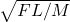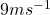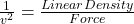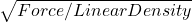A rectangular neoprene sheet has width W = 1.00 m and length L = 4.00 m. The two shorter edges are affixed to rigid steel bars that are used

Question

A rectangular neoprene sheet has width W = 1.00 m and length L = 4.00 m. The two shorter edges are affixed to rigid steel bars that are used to stretch the sheet taut and horizontal. The force applied to either end of the sheet is F = 81.0 N. The sheet has a total mass M = 4.00 kg. The left edge of the sheet is wiggled vertically in a uniform sinusoidal motion with amplitude A = 10.0 cm and frequency f = 1.00 Hz. This sends waves spanning the width of the sheet rippling from left to right. The right side of the sheet moves upward and downward freely as these waves complete their traversal.
(A) Derive an expression for the velocity with which the waves move along the sheet. Express your answer in terms of the variables F, L, and M.
(B) What is the value of this speed for the specified choices of these parameters? Express your answer with the appropriate units.

in progress 0
6 months 2021-07-15T10:22:06+00:00 1 Answers 5 views 0

(a)(b)Explanation:

(a)

The speed of the wave depends on the type of the material. Here we have neoprene sheet so the speed of the wave will depend on the linear density of neoprene sheet. Linear Density is defined as Mass per unit length of the material (as materials of same type can have different thickness). The symbol used for the Linear Density is .

μ = Mass of the sheet / Length of the sheet

The speed of the wave in such material can be be found by using the equation:(where v is speed)

The equation can be rearranged:

v =so,

v = sqrt(F/μ)

v = sqrt(F/ (M/L))

v =(answer)

(b)

Putting the values

F = 81 N

M = 4kg

L = 4m

v =v = 9m/s